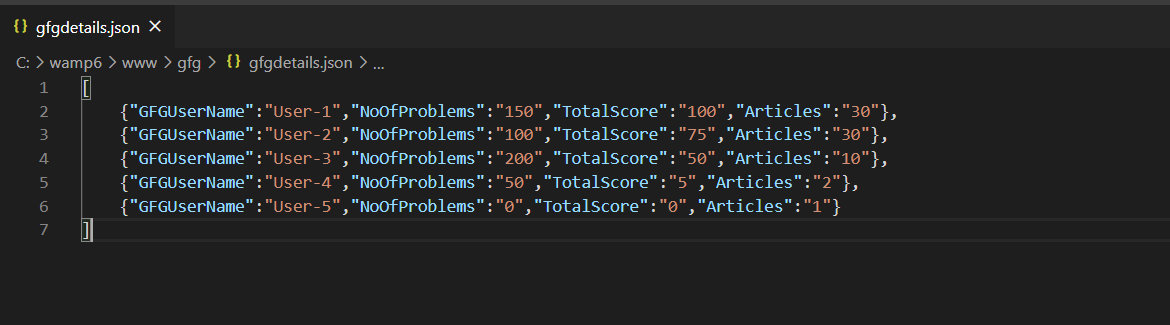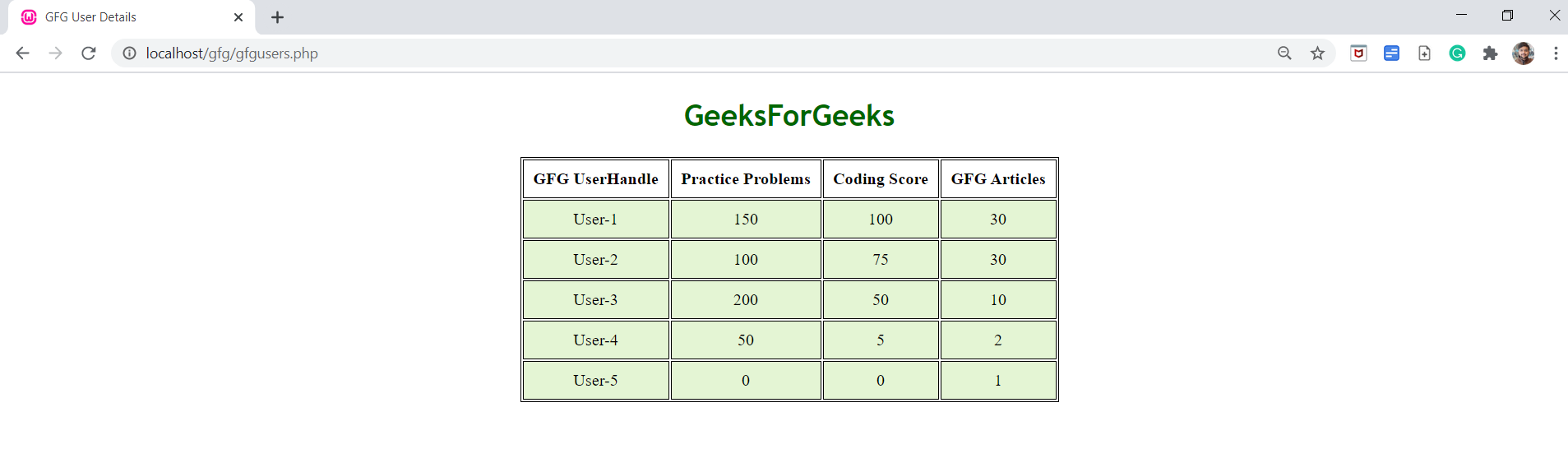Open in App
Not now

# How to fetch data from JSON file and display in HTML table using jQuery ?

• Difficulty Level : Expert
• Last Updated : 01 Jul, 2022

The task is to fetch data from the given JSON file and convert data into an HTML table.

Approach: We have a JSON file containing data in the form of an array of objects. In our code, we are using jQuery to complete our task. The jQuery code uses getJSON() method to fetch the data from the file’s location using an AJAX HTTP GET request. It takes two arguments. One is the location of the JSON file and the other is the function containing the JSON data. The each() function is used to iterate through all the objects in the array. It also takes two arguments. One is the data and the other one is the function containing the index and the element. An empty string is used to construct rows that contain the data from the JSON objects. The append() method is used to append the string containing rows in the table.

JSON file:Example:

 `<``html` `lang``=``"en"``> ` ` `  `<``head``> ` `    ``<``meta` `charset``=``"UTF-8"``> ` `    ``<``title``>GFG User Details ` ` `  `    ``` `    ``<``script` `src``= ` `"https://code.jquery.com/jquery-3.5.1.js"``> ` `    `` ` ` `  `    ``` `    ``<``style``> ` `        ``table { ` `            ``margin: 0 auto; ` `            ``font-size: large; ` `            ``border: 1px solid black; ` `        ``} ` ` `  `        ``h1 { ` `            ``text-align: center; ` `            ``color: #006600; ` `            ``font-size: xx-large; ` `            ``font-family: 'Gill Sans',  ` `                ``'Gill Sans MT', ' Calibri',  ` `                ``'Trebuchet MS', 'sans-serif'; ` `        ``} ` ` `  `        ``td { ` `            ``background-color: #E4F5D4; ` `            ``border: 1px solid black; ` `        ``} ` ` `  `        ``th, ` `        ``td { ` `            ``font-weight: bold; ` `            ``border: 1px solid black; ` `            ``padding: 10px; ` `            ``text-align: center; ` `        ``} ` ` `  `        ``td { ` `            ``font-weight: lighter; ` `        ``} ` `    `` ` ` ` ` `  `<``body``> ` `    ``<``section``> ` `        ``<``h1``>GeeksForGeeks ` ` `  `        ``` `        ``<``table` `id``=``'table'``> ` `            ``` `            ``<``tr``> ` `                ``<``th``>GFG UserHandle ` `                ``<``th``>Practice Problems ` `                ``<``th``>Coding Score ` `                ``<``th``>GFG Articles ` `            `` ` ` `  `            ``<``script``> ` `                ``\$(document).ready(function () { ` ` `  `                    ``// FETCHING DATA FROM JSON FILE ` `                    ``\$.getJSON("gfgdetails.json",  ` `                            ``function (data) { ` `                        ``var student = ''; ` ` `  `                        ``// ITERATING THROUGH OBJECTS ` `                        ``\$.each(data, function (key, value) { ` ` `  `                            ``//CONSTRUCTION OF ROWS HAVING ` `                            ``// DATA FROM JSON OBJECT ` `                            ``student += '<``tr``>'; ` `                            ``student += '<``td``>' +  ` `                                ``value.GFGUserName + ''; ` ` `  `                            ``student += '<``td``>' +  ` `                                ``value.NoOfProblems + ''; ` ` `  `                            ``student += '<``td``>' +  ` `                                ``value.TotalScore + ''; ` ` `  `                            ``student += '<``td``>' +  ` `                                ``value.Articles + ''; ` ` `  `                            ``student += ''; ` `                        ``}); ` `                         `  `                        ``//INSERTING ROWS INTO TABLE  ` `                        ``\$('#table').append(student); ` `                    ``}); ` `                ``}); ` `            `` ` `    `` ` ` ` ` `  ` `

Output:HTML is the foundation of webpages, is used for webpage development by structuring websites and web apps.You can learn HTML from the ground up by following this HTML Tutorial and HTML Examples.

jQuery is an open source JavaScript library that simplifies the interactions between an HTML/CSS document, It is widely famous with it’s philosophy of “Write less, do more”.
You can learn jQuery from the ground up by following this jQuery Tutorial and jQuery Examples.

My Personal Notes arrow_drop_up
Related Articles